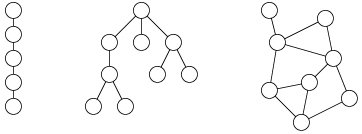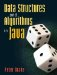# Graphs

So far we have dealt only with linear structures and trees. A linear structure can be considered a special case of a tree, where every node (except for the single leaf) has exactly one child. Just as trees generalize linear structures, graphs generalize trees (Figure 15-1). The key difference between trees and graphs is that, in a graph, there may be more than one path between two nodes.

Figure 15-1. A linear structure (left) is a special case of a tree (middle), which is in turn a special case of a graph (right).This chapter begins with discussions of graph terminology (Section 15.1), representation (Section 15.2), and traversal (Section 15.3). The remaining sections present several algorithms related to graphs. An incredible variety of computational problems can be phrased in terms of graphs. For example:

• Consider a set of tasks in a complicated cooking or industrial fabrication process. Some of the tasks have others as prerequisites. In what order can the tasks be performed? This is the topological sorting problem, addressed in Section 15.4.
• What is the shortest driving route from Los Angeles to Chicago? Section 15.5 covers algorithms for finding shortest paths.
• Given a set of computers in various locations in a building, how can they be connected with the least amount of cable? This is the problem of finding a minimum spanning tree, discussed in Section 15.6.Data Structures and Algorithms in Java
ISBN: 0131469142
EAN: 2147483647
Year: 2004
Pages: 216
Authors: Peter Drake